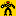## 22 October 2010

### Math versus Tanks

This story at Wired tells how statistical methods were used to estimate German tank production during World War II:The Germans had given each tank a production number, and each captured tank provided information about how many more tanks were in the German army. If you capture 10 tanks, and the highest production number among these is #800, then you know there are at least 790 other tanks out there. If you can assume these tanks were captured more or less at random, then it is unlikely that tank #800 just happened be be among these 10, and the 10 numbers observed ought to be spread out unevenly between 1 and 800. The total number of tank should be something just a bit more than the highest number you happen to know.
Allied intelligence noticed each captured tank had a unique serial number. With careful observation, the Allies were able to determine the serial numbers had a pattern denoting the order of tank production. Using this data, the Allies created a mathematical model to determine the rate of German tank production. They used it to estimate that the Germans produced 255 tanks per month between the summer of 1940 and the fall of 1942.
Turns out the serial-number methodology was spot on. After the war, internal German data put der Führer’s production at 256 tanks per month — one more than the estimate.
That's a very good estimate. Are the statistics here really that good, or did they just get lucky? Using the formulas in the article and my example above with 800 the highest number observed out of 10 captured, the estimate of total number is equal to 800+800/10 - 1 = 879, with a margin of error (~1 standard deviations) of plus-or-minus 88, or about 10% of the estimate That's not so bad given that I only "sampled" 10 tanks, but there is a lot of room for error. It gets better as the sample gets larger though. It turns out the variability of this estimate is inversely proportional to the sample size, 10 tanks give a standard deviation of about 1/10, or 10%. Bump that up to 100 tanks and the standard deviation is 1/100, or 1%, and now the estimate is precise enough that your military planners won't care about the error.

This isn't the only example of statistics in war; many basic quality control methods were originally devised as part of the effort for World War II.